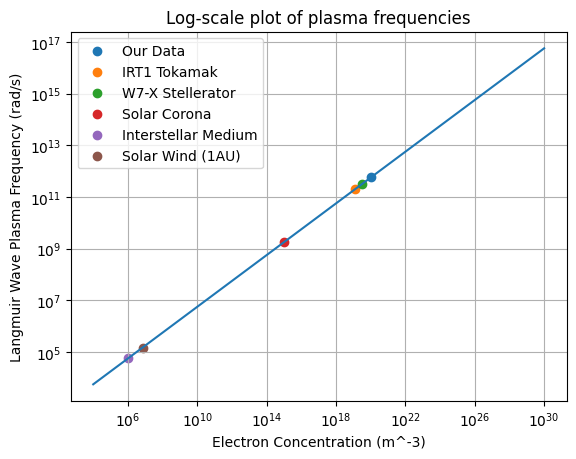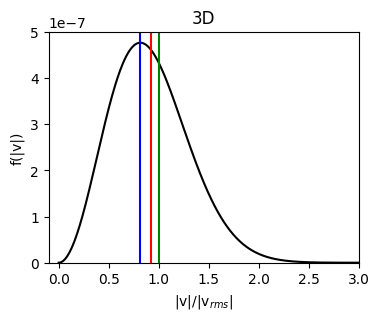# Speed Plasma Parameters (plasmapy.formulary.speeds)

Functions to calculate fundamental plasma speed parameters.

## Functions

 Alfven_speed(B, density[, ion, z_mean]) Calculate the Alfvén speed. ion_sound_speed(T_e, T_i, ion[, n_e, k, ...]) Return the ion sound speed for an electron-ion plasma. kappa_thermal_speed(T, kappa, particle[, method]) Return the most probable speed for a particle within a Kappa distribution. thermal_speed(T, particle[, method, mass, ndim]) Calculate the speed of thermal motion for particles with a Maxwellian distribution. thermal_speed_coefficients(method, ndim) Get the thermal speed coefficient corresponding to the desired thermal speed definition.

## Aliases

PlasmaPy provides short-named (alias) versions of the most common plasma functionality. These aliases are only given to functionality where there is a common lexicon in the community, for example has the alias . All aliases in PlasmaPy are denoted with a trailing underscore _.

 cs_(T_e, T_i, ion[, n_e, k, gamma_e, ...]) Alias to ion_sound_speed. va_(B, density[, ion, z_mean]) Alias to Alfven_speed. vth_(T, particle[, method, mass, ndim]) Alias to thermal_speed(). vth_kappa_(T, kappa, particle[, method]) Alias to kappa_thermal_speed.

## Lite-Functions

Much of PlasmaPy’s functionality incorporates Astropy units for user convenience and to mitigate calculation errors from inappropriate units, but this comes at the sacrifice of speed. While this penalty is not significant for typical use, it can become substantial during intensive numerical calculations. Lite-functions are introduced for the specific case where speed matters, but [USER NOTICE] this comes with the reduction of safeguards so a user needs to know what they are doing! For additional details look to the glossary entry for .

 thermal_speed_lite(T, mass, coeff) The "lite-function" version of thermal_speed.

## Examples